Author: Oscar Cronquist Article last updated on September 30, 2022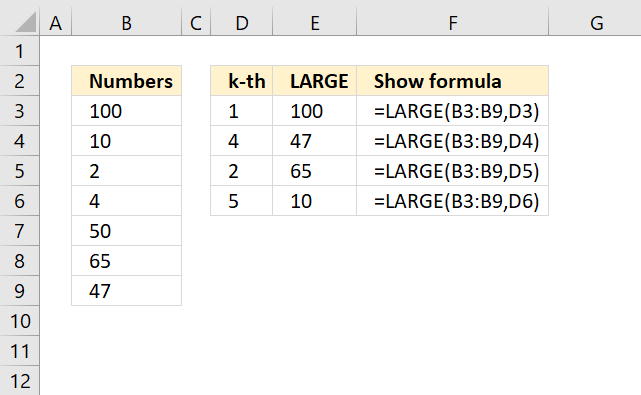The LARGE function calculates the k-th largest value from an array of numbers.

Use the LARGE function, for example, to extract the highest number, second highest, and third highest from a LARGE range of numbers.

LARGE(array, k)

## 2. LARGE Function Arguments

 array Required. Group of numbers for which you want to calculate the k-th largest value. k Required. The position in the group of numbers to return, sorted from the largest.

## 3. LARGE function - example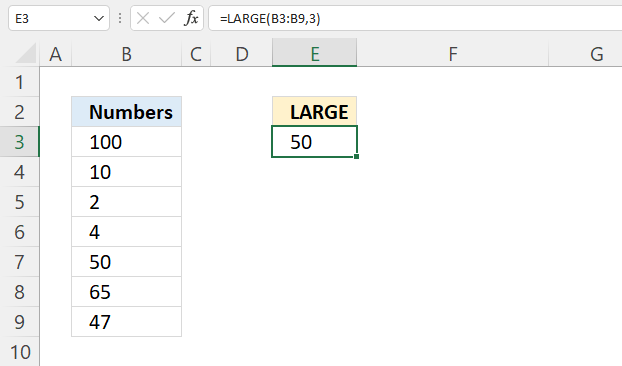The example demonstrated in cell E3 extracts the third-largest number in cell range B3:B9.

Formula in cell C3:

=LARGE(B3:B9, 3)

LARGE(array, k)

array - B3:B9
k - 3

LARGE(B3:B9, 3)

becomes

LARGE({100; 10; 2; 4; 50; 65; 47}, 3)

and returns 50. 50 is the third-largest number in B3:B9. Only 100 and 65 are larger.

## 4. LARGE function - based on a condition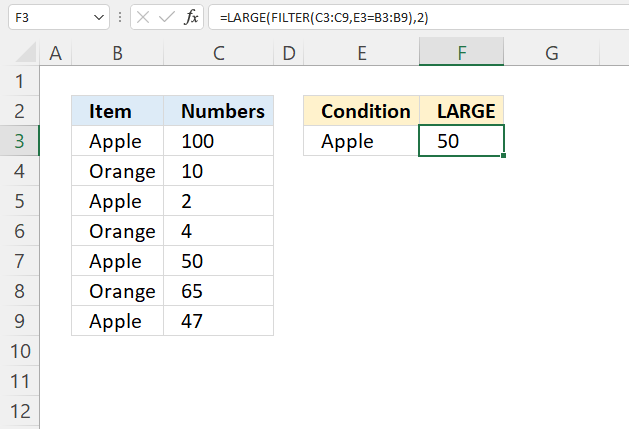The formula in cell F3 extracts the second largest number in cell range C3:C9 if the corresponding value on the same row in cell range B3:B9 is equal to the condition specified in cell E3.

Formula in cell F3:

=LARGE(FILTER(C3:C9, E3=B3:B9), 2)

### 4.1 Explaining formula

#### Step 1 - Logical test

The equal sign compares values in an Excel formula, the result is a boolean value TRUE or FALSE.

E3=B3:B9

becomes

"Apple"={"Apple"; "Orange"; "Apple"; "Orange"; "Apple"; "Orange"; "Apple"}

and returns

{TRUE; FALSE; TRUE; FALSE; TRUE; FALSE; TRUE}

#### Step 2 - Filter values based on logical test

The FILTER function extract values/rows based on a condition or criteria.

FILTER(arrayinclude, [if_empty])

FILTER(C3:C9,E3=B3:B9)

becomes

FILTER(C3:C9,{TRUE; FALSE; TRUE; FALSE; TRUE; FALSE; TRUE})

becomes

FILTER({100; 10; 2; 4; 50; 65; 47},{TRUE; FALSE; TRUE; FALSE; TRUE; FALSE; TRUE})

and returns

{100; 2; 50; 47}

#### Step 3 - Calculate the second largest value

LARGE(FILTER(C3:C9,E3=B3:B9),2)

becomes

LARGE({100; 2; 50; 47},2)

and returns 50.

## 5. LARGE function - based on criteria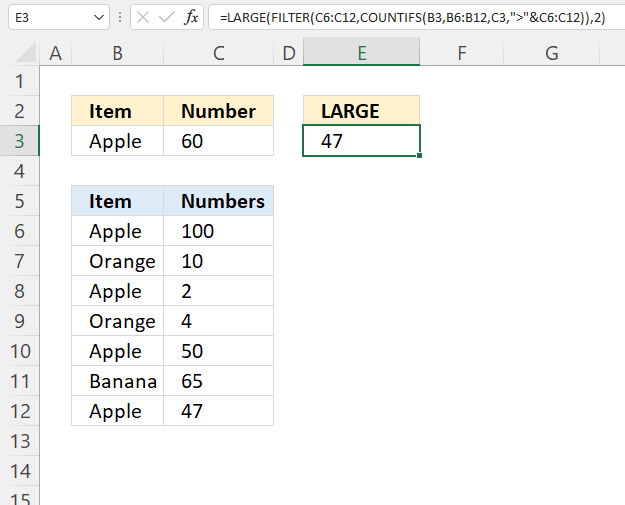The formula in cell E3 extracts the second largest number in cell range C6:C12 if two conditions are met, the first condition is specified in cell B3 and the second in cell C3.

Note, the second condition is if a number is smaller than 60.

Formula in cell E3:

=LARGE(FILTER(C6:C12, COUNTIFS(B3, B6:B12, C3, ">"&C6:C12)), 2)

### 5.1 Explaining formula

#### Step 1 - Check criteria

The COUNTIFS function calculates the number of cells across multiple ranges that equals all given conditions.

COUNTIFS(criteria_range1criteria1, [criteria_range2criteria2]…)

Note the less than character in the last argument.

COUNTIFS(B3, B6:B12, C3, ">"&C6:C12)

returns

{0; 0; 1; 0; 1; 0; 1}.

Rows 8, 10 and 12 meet both conditions, see the image above.

#### Step 2 - Filter list

The FILTER function extract values/rows based on a condition or criteria.

FILTER(arrayinclude, [if_empty])

FILTER(C6:C12, COUNTIFS(B3, B6:B12, C3, ">"&C6:C12))

becomes

FILTER(C6:C12, {0; 0; 1; 0; 1; 0; 1})

becomes

FILTER({100; 10; 2 ;4; 50; 65; 47}, {0; 0; 1; 0; 1; 0; 1})

and returns {2; 50; 47}.

#### Step 3 - Calculate the second largest value

LARGE(FILTER(C6:C12, COUNTIFS(B3, B6:B12, C3, ">"&C6:C12)), 2)

becomes

LARGE({2; 50; 47}, 2)

and returns 47.

## 6. LARGE function - based on a list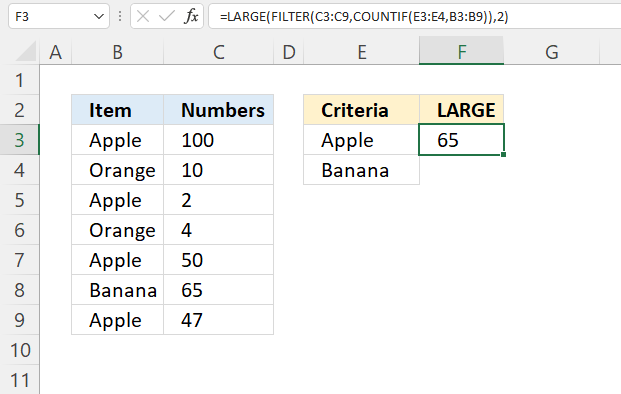The formula in cell F3, in the image above, extracts the second largest number in cell range C3:C9 if the value on the same row in B3:B9 meets any of the conditions specified in cells E3 and E4.

In other words, OR logic applied to a single column.

Formula in cell F3:

=LARGE(FILTER(C3:C9, COUNTIF(E3:E4, B3:B9)), 2)

### 6.1 Explaining formula

#### Step 1 - Compare the list to items

The COUNTIF function counts the number of cells that meets a condition.

COUNTIF(rangecriteria)

COUNTIF(E3:E4, B3:B9)

becomes

COUNTIF({"Apple"; "Banana"},{"Apple"; "Orange"; "Apple"; "Orange"; "Apple"; "Banana"; "Apple"})

and returns

{1; 0; 1; 0; 1; 1; 1}

#### Step 2 - Filter values based on logical test

The FILTER function extract values/rows based on a condition or criteria.

FILTER(arrayinclude, [if_empty])

FILTER(C3:C9,COUNTIF(E3:E4, B3:B9))

becomes

FILTER(C3:C9,{1; 0; 1; 0; 1; 1; 1})

becomes

FILTER({100; 10; 2; 4; 50; 65; 47}, {1; 0; 1; 0; 1; 1; 1})

and returns

{100; 2; 50; 65, 47}.

#### Step 3 - Calculate the second largest value

LARGE(FILTER(C3:C9,E3=B3:B9),2)

becomes

LARGE({100; 2; 50; 65, 47},2)

and returns 65.

## 7. LARGE function - multiple source ranges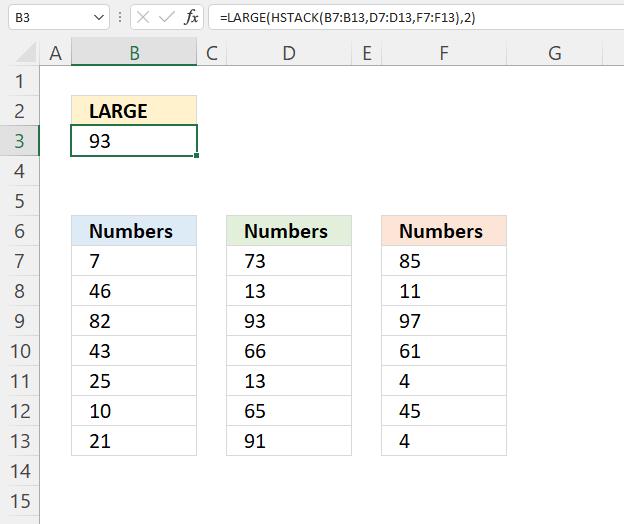The formula in cell B3 extracts the second largest number from three different nonadjacent cell ranges, in this example located on the same worksheet. The formula works fine even if they are on different worksheets.

Formula in cell B3:

=LARGE((B7:B13,D7:D13,F7:F13),2)

### 7.1 Explaining formula

#### Step 1 - Join cell ranges

The parentheses and commas let you join cell ranges in the LARGE function, this doesn't work in every function. However, the LARGE and SMALL function works.

(B7:B13,D7:D13,F7:F13)

becomes

({7; 46; 82; 43; 25; 10; 21},{73; 13; 93; 66; 13; 65; 91},{85; 11; 97; 61; 4; 45; 4})

and returns

{7,73,85; 46,13,11; 82,93,97; 43,66,61; 25,13,4; 10,65,45; 21,91,4}.

#### Step2 - Second largest value in the array

LARGE((B7:B13,D7:D13,F7:F13),2)

becomes

LARGE({7,73,85; 46,13,11; 82,93,97; 43,66,61; 25,13,4; 10,65,45; 21,91,4},2)

and returns 93. Only 97 is larger.

## 8. LARGE function - based on a text string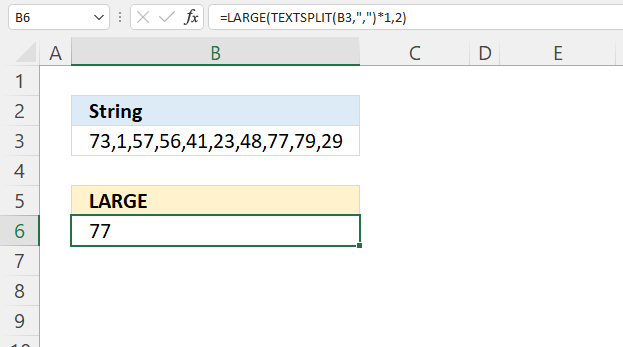The formula in cell B6 splits the string specified in cell B3 into an array, then extracts the second largest number in the array.

Formula in cell B6:

=LARGE(TEXTSPLIT(B3,",")*1,2)

### 8.1 Explaining formula

#### Step 1 - Split string

The TEXTSPLIT function lets you split a string into an array across columns and rows based on delimiting characters.

TEXTSPLIT(Input_Textcol_delimiter, [row_delimiter], [Ignore_Empty])

TEXTSPLIT(B3,",")

becomes

TEXTSPLIT("73,1,57,56,41,23,48,77,79,29",",")

and returns

{"73", "1", "57", "56", "41", "23", "48", "77", "79", "29"}.

#### Step 2 - Convert to numbers

The asterisk lets you multiply values in an Excel formula, it also lets you convert "text" numbers to regular numbers. The numbers in the array above have double quotes, these will be removed.

TEXTSPLIT(B3,",")*1

becomes

{"73", "1", "57", "56", "41", "23", "48", "77", "79", "29"}*1

and returns

{73, 1, 57, 56, 41, 23, 48, 77, 79, 29}.

#### Step 3 - Extract the second largest number in the array

LARGE(TEXTSPLIT(B3,",")*1,2)

becomes

LARGE({"73", "1", "57", "56", "41", "23", "48", "77", "79", "29"}, 2)

and returns 77. Only 79 is larger.

## 9. LARGE function - calculate an average of the three largest numbers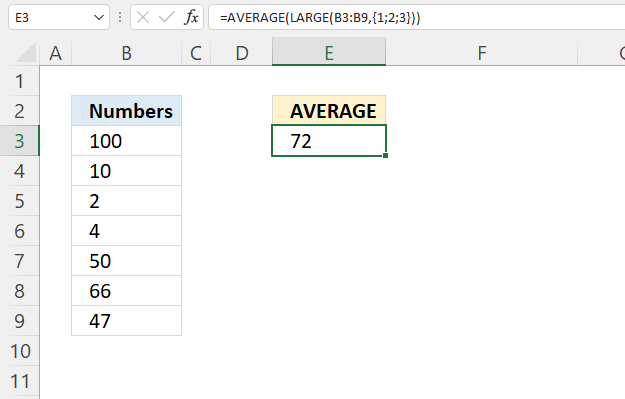The formula in cell E3 extracts the three largest numbers in cell range B3:B9, then calculates an average based on these three numbers.

Formula in cell range E3:

=AVERAGE(LARGE(B3:B9,{1;2;3}))

### 9.1 Explaining formula

#### Step 1 - Extract the three largest numbers

The LARGE function allows you to extract multiple values if you use an array of numbers in the second argument.

LARGE(B3:B9,{1;2;3})

becomes

LARGE({100; 10; 2; 4; 50; 66; 47},{1; 2; 3})

and returns

{100; 66; 50}.

#### Step 2 -  Calculate an average

The AVERAGE function calculates the average of numbers in a cell range or array.

AVERAGE(number1[number2], ...)

AVERAGE(LARGE(B3:B9,{1; 2; 3}))

becomes

AVERAGE({100; 66; 50})

and returns 72. 100 + 66 + 50 = 216. 216/3 equals 72.

## 10. How to extract the k-th largest number in a 3D range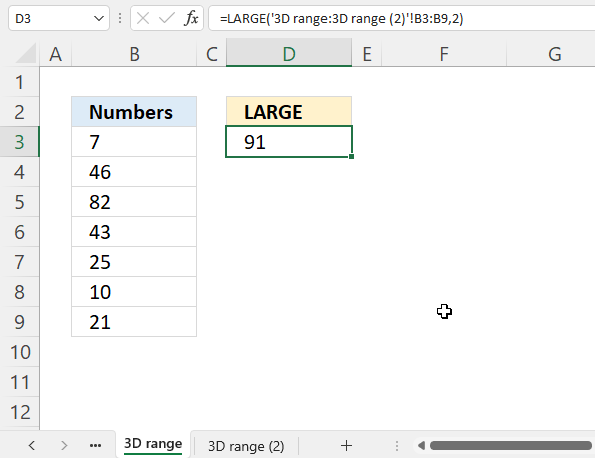This example shows how to extract the k-th largest number in multiple worksheets. The data must be in the same cell range throughout all worksheets. For example, the image above demonstrates two cell ranges B3:B9 in worksheets '3D range' and '3D range (2)'.

Here is how to enter the LARGE function using 3D ranges:

1. Doublepress with left mouse button on a cell.
2. Type =LARGE(
3. Press and hold SHIFT key.
4. Select the remaining worksheets, in this case '3D range (2)' with the mouse.
5. Select cell range B3:B9 with the mouse.
6. Type the ending parentheses.
7. Press Enter.

The formula looks like this;

=LARGE('3D range:3D range (2)'!B3:B9,2)

Section 7 demonstrates how to get the k-th largest value from multiple cell ranges, this works fine with multiple worksheets as well and they don't need to be located on the same cell range. This can however be tedious to enter if many cell ranges are used.